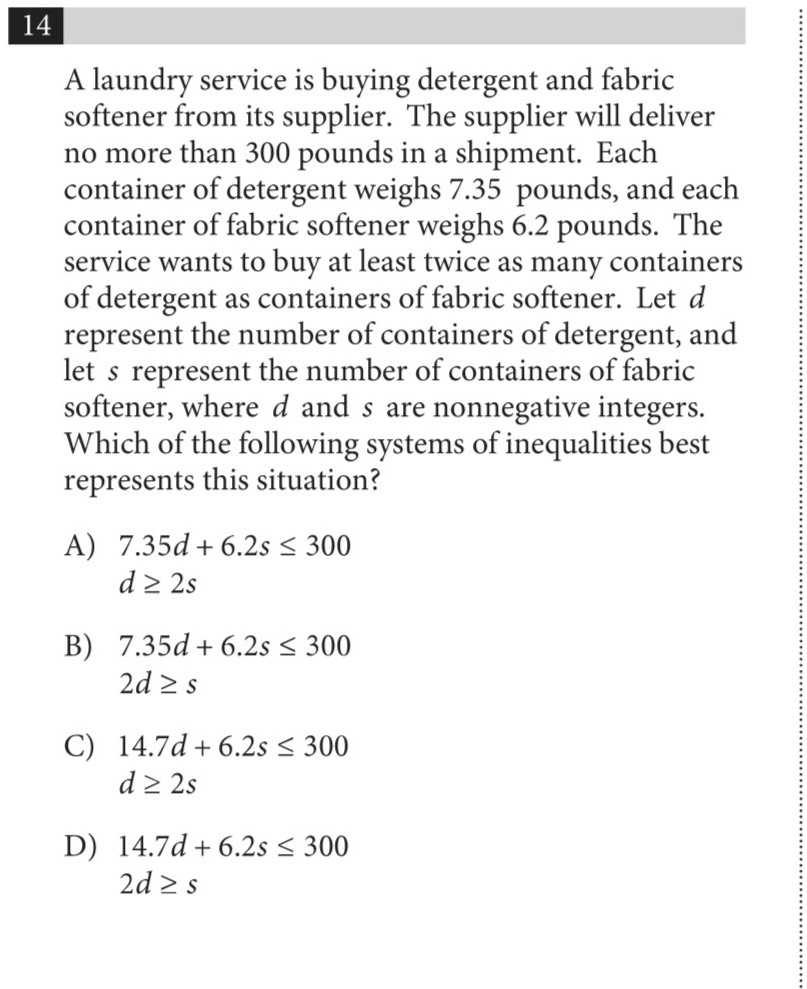### ¿Todavía tienes preguntas de matemáticas?

Pregunte a nuestros tutores expertos
Algebra
PreguntaA laundry service is buying detergent and fabric softener from its supplier. The supplier will deliver no more than $$300$$ pounds in a shipment. Each container of detergent weighs $$7.35$$ pounds, and each container of fabric softener weighs $$6.2$$ pounds. The service wants to buy at least twice as many containers of detergent as containers of fabric softener. Let $$d$$ represent the number of containers of detergent, and let $$s$$ represent the number of containers of fabric softener, where $$d$$ and $$s$$ are nonnegative integers. Which of the following systems of inequalities best represents this situation? A) $$7.35 d + 6.2 s \leq 300$$

$$d \geq 2 s$$

B) $$7.35 d + 6.2 s \leq 300$$

$$2 d \geq s$$

C) $$14.7 d + 6.2 s \leq 300$$

$$d \geq 2 s$$

D) $$14.7 d + 6.2 s \leq 300$$

$$2 d \geq s$$